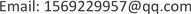1三峡大学计算机与信息学院，湖北 宜昌

2三峡大学理学院，湖北 宜昌1. 问题分析

2. 模型的建立2.1. 模型的准备

2.2. 模型的建立2.2.1. 行车安全指标体系的建立

1) 运输车辆质量大，运输时间长，车速因素对交通安全有重要影响，一般运输车辆的限速为80 km/h，记车速超过限速的时间比例为：

η = T > 80 T

2) 车速标准差表现为速度的离散程度，车速分布得越离散，发生事故的概率越大，即为：

v s = 1 n ∑ m = 1 n ( v m − v a ) 2

3) 通过对方向盘转角的离散程度分析还可以对驾驶人的操控的稳定性进行评估。方向盘转角的离散程度可以用方向盘转角的标准差来判断：

S t e e r i n g D e g = 1 N − 1 ∑ i = 1 N ( deg i − deg ¯ ) 2

1) 平面线性与行车安全

2) 平曲线

R min = v 2 127 ( μ + i )

1) 路面宽度与行车安全。总体来看事故率的发生于路面宽度基本是线性关系的，路面越窄，事故率越小

2) 中间带宽度与行车安全，根据已经统计的结果显示如表1：

Situation after the separation belt is use

1) 护栏与行车安全。半刚性护栏是通过车辆与护栏间的摩擦，车辆与地面间的摩擦，护栏产生一定的弹性变形来吸收碰撞能量，延长碰撞过程的作用时间。可以达到降低车速，使失控车辆改变行驶方向的效果，从而保证驾驶人的安全和安全事故发生的概率。

2) 防眩设施。防眩设施是指分布在中央分隔带上用于清除汽车前照灯夜间眩光影响的安全设施。他的分布根据地理条件等方面考虑，评价这个指标时可以按照防眩设施完备率，防眩高度和连续率等指标进行，防眩设施完备率用公式可以表示为：

ϕ = r s × 100 %

3) 交通标志。在道路上的交通标志对驾驶员对道路的观察及时作出判断起到很大的作用。交通标志旨在通过对驾驶员适时，准确的诱导，充分发挥道路安全的效能。评价时可以按照标志完备率，限速标志最小间距率，不合理标志使用率，信息过载率等指标进行评价。

Q = V ⋅ K

1) 雨雪雾天气分析

Daily precipitation and risk leve

Stratified analysis of daily mean temperatur

[-, 2)0.213
[2, 12)0.512
[12, 26)0.118
[26, +)0.157

Daily minimum visibility stratification analysis result

[0, 200)0.211
[200, 500)0.495
[500, 1000)0.201
[1000, +)0.093

y = b 0 ( 1 ) + b 1 ( 1 ) ⋅ x 1

y = b 0 ( 2 ) + b 1 ( 2 ) ⋅ x 1 + b 2 ( 2 ) ⋅ x 2

2.2.2. 节能指标体系的建立

1) 驾驶员操作。当驾驶员在行驶过程中反复出现怠速预热，空挡滑行，紧急制动，车速的不稳定性操作时。这在油耗上是一个很大的浪费。评价车辆是否节能时，可以当做驾驶员操作的评价指标。分别记录出现这些行为的次数S。

2) 环境温度和湿度的影响。和常温相比，当环境温度为−10度时，燃油消耗量增加高达45%。0度时的燃油消耗量增加了22%。而且湿度对燃油经济性的影响也很大，高于常温时，影响相对较少，高于0度时，湿度30%比湿度80%时节油15%。

3) 道路条件的影响。道路特征对运输车节能主要表现在各种路面条件下滚动阻力系数的差异。其中

F f = G a ⋅ g ⋅ f

Ff为滚动阻力，运输车质量一定，在不同的滚动阻力下行驶得油耗也是不同的。

2.2.3. 效率指标体系的建立

1) 工作车时利用率。统计车辆在线路上的行驶车时与线路工作车时之比，线路工作车时由行驶车时和线路上的停歇车时组成。即为：

λ = t t + t ′ × 100 %

2) 运送速度。车辆在运送时间内的平均速度，只计算起运点出发到终运点到达时刻。即为：

v ¯ = S T

3) 工作率。统计期内工作日车与总车日之比，营运车辆一天内只要有工作即为一个工作车日。

w i = α i β i ∑ i = 1 4 α i β

1) AHP法确定权重，如表5所示。

AHP Method to determine the weigh

1表示Bi与Bj两个因素同等重要
3表示Bi比Bj稍重要
5表示Bi比Bj明显重要
7表示Bi比Bj重要得多
2、3、6表示相邻两标度的中间值

C . I . = λ ( A ) − n n − 1

C . R . = C . I . / R . I .

2) 熵值法确定权重的大小

a) 选取n个评价对象，m个评价指标，则xij为第i辆运输车的第j个指标的数值其中

( i = 1 , 2 , ⋯ n ; j = 1 , 2 , 3 , ⋯ m )

b) 指标的归一化处理：异质指标同质化

x i j ' = x i j − min { x i j , ⋯ x n j } max { x i j , ⋯ x n j } − min { x i j , ⋯ x n j } x i j ' = max { x i j , ⋯ x n j } − x i j max { x i j , ⋯ x n j } − min { x i j , ⋯ x n j }

c) 计算第j项指标下第i辆运输车占该指标的比重：

p i j = x i j ∑ i = 1 n x i j ( i = 1 , ⋯ n ; j = 1 , ⋯ m )

d) 计算j项指标的熵值：

e j = − k ∑ i = 1 n p i j ln ( p i j )

e) 计算信息熵冗余度：

d j = 1 − e j

f) 计算各项指标的权值：

β j = d j ∑ j = 1 m d j

A i = [ a i 1 , a i 2 , ⋯ a i n ]

B i = A i ⋅ R ˜ i

K = [ u ˜ 1 , u ˜ 2 , ⋯ u ˜ n ]

B = A ⋅ R

Evaluation standard tabl

3. 模型的求解

Y = 2.132 R − 1.167 T + 192.412

AHP-entropy method to determine weight

4. 结语